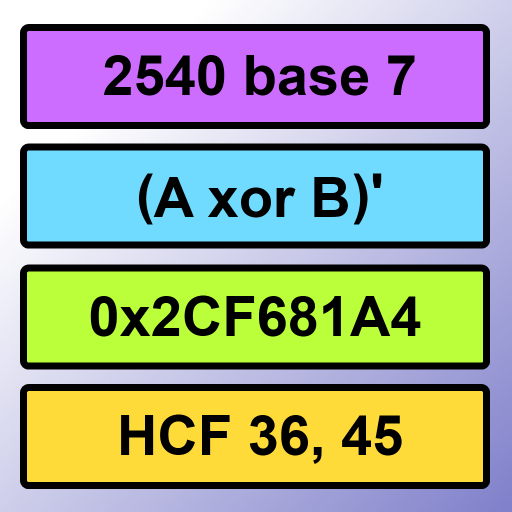CalculatorLogical Calculator

A 5 in 1 whole number calculator which provides useful functionality for those who are interested in computing. Each screen has a help mode which provides a description of each function. Inaddition, values can be stored and shared across screens. A detailed list of each screen/calculator is given below.

Decimal Calculator
• 32-bit and signed 31-bit modes
• Conversions from decimal to bases 2-9 and hexadecimal
• Logical Operators: AND, OR
• Random Number Generator
• Power and Mod functions
Binary Calculator
• 4-bit, 8-bit and 16-bit modes.
• Logical Operators: AND, OR, XOR, NAND, NOR, XNOR and NOT
• Two's Complment Operation
• Random Number Generator
• Left Shift and Right Shift Functions
• Results show as Boolean Expressions in the 4-bit mode